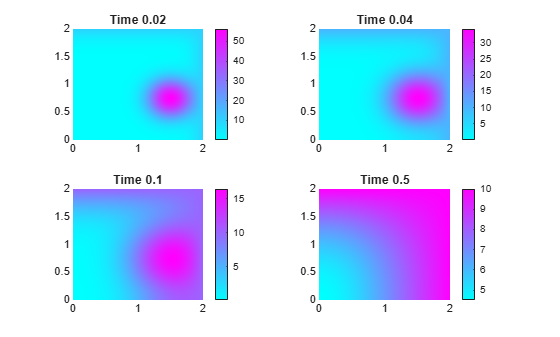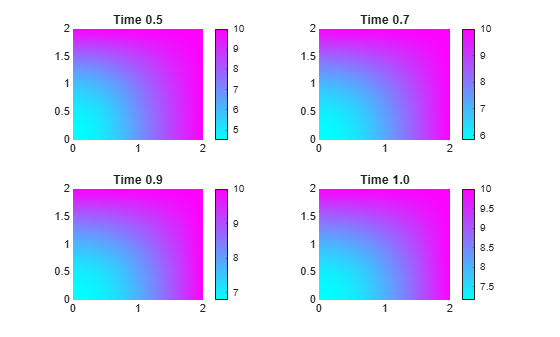# setInitialConditions

Give initial conditions or initial solution

## Syntax

``setInitialConditions(model,u0)``
``setInitialConditions(model,u0,ut0)``
``setInitialConditions(___,RegionType,RegionID)``
``setInitialConditions(model,results)``
``setInitialConditions(model,results,iT)``
``ic = setInitialConditions(___)``

## Description

example

````setInitialConditions(model,u0)` sets initial conditions in `model`. Use this syntax for stationary nonlinear problems or time-dependent problems where the time derivative is first order. NoteInclude geometry in `model` before using `setInitialConditions`. ```

example

````setInitialConditions(model,u0,ut0)` use this syntax for time-dependent problems where a time derivative is second order, such as a hyperbolic problem.```

example

````setInitialConditions(___,RegionType,RegionID)` sets initial conditions on a geometry region using any of the arguments in the previous syntaxes.```

example

````setInitialConditions(model,results)` sets the initial guess for stationary nonlinear problems using the solution `results` from a previous analysis on the same geometry and mesh. The initial derivative for stationary problems is 0.```

example

````setInitialConditions(model,results,iT)` sets the initial conditions for time-dependent problems using the solution `results` corresponding to the solution time index `iT`. If you do not specify the time index `iT`, `setInitialConditions` uses the last solution time in `results`.```
````ic = setInitialConditions(___)` returns a handle to the initial conditions object.```

## Examples

collapse all

Create a PDE model, import geometry, and set the initial condition to 50 on the entire geometry.

```model = createpde(); importGeometry(model,'BracketWithHole.stl'); setInitialConditions(model,50);```

Set different initial conditions for each component of a system of PDEs.

Create a PDE model for a system with five components. Import the `Block.stl` geometry.

```model = createpde(5); importGeometry(model,'Block.stl');```

Set the initial conditions for each component to twice the component number.

```u0 = [2:2:10]'; setInitialConditions(model,u0)```
```ans = GeometricInitialConditions with properties: RegionType: 'cell' RegionID: 1 InitialValue: [5x1 double] InitialDerivative: [] ```

Set different initial conditions on each portion of the L-shaped membrane geometry.

Create a model, set the geometry function, and view the subdomain labels.

```model = createpde(); geometryFromEdges(model,@lshapeg); pdegplot(model,'FaceLabels','on') axis equal ylim([-1.1,1.1])```Set subdomain 1 to initial value -1, subdomain 2 to initial value 1, and subdomain 3 to initial value 5.

```setInitialConditions(model,-1); setInitialConditions(model,1,'Face',2); setInitialConditions(model,5,'Face',3);```

The initial setting applies to the entire geometry. The subsequent settings override the initial settings for regions 2 and 3.

Set initial conditions for the L-shaped membrane geometry to be ${x}^{2}+{y}^{2}$, except in the lower left square where it is ${x}^{2}-{y}^{4}$.

```model = createpde(); geometryFromEdges(model,@lshapeg); pdegplot(model,'FaceLabels','on') axis equal ylim([-1.1,1.1])```Set the initial conditions to ${x}^{2}+{y}^{2}$.

```initfun = @(location)location.x.^2 + location.y.^2; setInitialConditions(model,initfun);```

Set the initial conditions on region 2 to ${x}^{2}-{y}^{4}$. This setting overrides the first setting because you apply it after the first setting.

```initfun2 = @(location)location.x.^2 - location.y.^4; setInitialConditions(model,initfun2,'Face',2);```

Hyperbolic equations have nonzero `m` coefficient, so you must set both the `u0` and `ut0` arguments.

Import the `Block.stl` to a PDE model with N = 3 components.

```model = createpde(3); importGeometry(model,'Block.stl');```

Set the initial condition value to be 0 for all components. Set the initial derivative.

`$ut0=\left[\begin{array}{c}4+\frac{x}{{x}^{2}+{y}^{2}+{z}^{2}}\\ 5-\mathrm{tanh}\left(z\right)\\ 10\frac{y}{{x}^{2}+{y}^{2}+{z}^{2}}\end{array}\right]$`

To create this initial gradient, write a function file, and ensure that the function is on your MATLAB path.

`function ut0 = ut0fun(location)`

`M = length(location.x);`

`ut0 = zeros(3,M);`

`denom = location.x.^2+location.y.^2+location.z.^2;`

`ut0(1,:) = 4 + location.x./denom;`

`ut0(2,:) = 5 - tanh(location.z);`

`ut0(3,:) = 10*location.y./denom;`

`end`

Set the initial conditions.

`setInitialConditions(model,0,@ut0fun)`
```ans = GeometricInitialConditions with properties: RegionType: 'cell' RegionID: 1 InitialValue: 0 InitialDerivative: @ut0fun ```

Set initial conditions using the solution from a previous analysis on the same geometry and mesh.

Create and view the geometry: a square with a circular subdomain.

```% Square centered at (1,1), circle centered at (1.5,0.5). rect1 = [3;4;0;2;2;0;0;0;2;2]; circ1 = [1;1.5;.75;0.25]; % Append extra zeros to the circle; circ1 = [circ1;zeros(length(rect1)-length(circ1),1)]; gd = [rect1,circ1]; ns = char('rect1','circ1'); ns = ns'; sf = 'rect1+circ1'; [dl,bt] = decsg(gd,sf,ns); pdegplot(dl,'EdgeLabels','on','FaceLabels','on') axis equal ylim([-0.1,2.1])```Include the geometry in a PDE model, set boundary and initial conditions, and specify coefficients.

```model = createpde(); geometryFromEdges(model,dl); % Set boundary conditions that the upper % and left edges are at temperature 10. applyBoundaryCondition(model,'dirichlet', ... 'Edge',[2,3],'u',10); % Set initial conditions that the square region % is at temperature 0, % and the circle is at temperature 100. setInitialConditions(model,0); setInitialConditions(model,100,'Face',2); specifyCoefficients(model,'m',0,... 'd',1,... 'c',1,... 'a',0,... 'f',0);```

Solve the problem for times 0 through 1/2 in steps of 0.01.

```generateMesh(model,'Hmax',0.05); tlist = 0:0.01:0.5; results = solvepde(model,tlist);```

Plot the solution for times 0.02, 0.04, 0.1, and 0.5.

```sol = results.NodalSolution; subplot(2,2,1) pdeplot(model,'XYData',sol(:,3)) title('Time 0.02') subplot(2,2,2) pdeplot(model,'XYData',sol(:,5)) title('Time 0.04') subplot(2,2,3) pdeplot(model,'XYData',sol(:,11)) title('Time 0.1') subplot(2,2,4) pdeplot(model,'XYData',sol(:,51)) title('Time 0.5')```Now, resume the analysis and solve the problem for times from 1/2 to 1. Use the previously obtained solution for time 1/2 as an initial condition. Since 1/2 is the last element in `tlist`, you do not need to specify the solution time index. By default, `setInitialConditions` uses the last solution index.

`setInitialConditions(model,results)`
```ans = NodalInitialConditions with properties: InitialValue: [7289x1 double] InitialDerivative: [] ```

Solve the problem for times 1/2 through 1 in steps of 0.01.

```tlist1 = 0.5:0.01:1.0; results1 = solvepde(model,tlist1);```

Plot the solution for times 0.5, 0.7, 0.9, and 1.

```sol1 = results1.NodalSolution; figure subplot(2,2,1) pdeplot(model,'XYData',sol1(:,1)) title('Time 0.5') subplot(2,2,2) pdeplot(model,'XYData',sol1(:,21)) title('Time 0.7') subplot(2,2,3) pdeplot(model,'XYData',sol1(:,41)) title('Time 0.9') subplot(2,2,4) pdeplot(model,'XYData',sol1(:,51)) title('Time 1.0')```To use the previously obtained solution for a particular solution time instead of the last one, specify the solution time index as a third parameter of `setInitialConditions`. For example, use the solution at time 0.2, which is the 21st element in `tlist`.

`setInitialConditions(model,results,21)`
```ans = NodalInitialConditions with properties: InitialValue: [7289x1 double] InitialDerivative: [] ```

Solve the problem for times 0.2 through 1 in steps of 0.01.

```tlist2 = 0.2:0.01:1.0; results2 = solvepde(model,tlist2);```

## Input Arguments

collapse all

PDE model, specified as a `PDEModel` object.

Example: `model = createpde`

Initial conditions, specified as a scalar, a column vector of length N, or a function handle. N is the size of the system of PDEs. See Equations You Can Solve Using PDE Toolbox.

• Scalar — Use it to represent a constant initial value for all solution components throughout the domain.

• Column vector — Use it to represent a constant initial value for each of the N solution components throughout the domain.

• Function handle — Use it to represent the initial conditions as a function of position. The function must be of the form

`u0 = initfun(location)`

Solvers pass `location` as a structure with fields `location.x`, `location.y`, and, for 3-D problems, `location.z`. `initfun` must return a matrix `u0` of size N-by-`M`, where ```M = length(location.x)```.

Example: `setInitialConditions(model,10)`

Data Types: `double` | `function_handle`
Complex Number Support: Yes

Initial condition for time derivative, specified as a scalar, a column vector of length N, or a function handle. N is the size of the system of PDEs. See Equations You Can Solve Using PDE Toolbox. You must specify `ut0` when there is a nonzero second-order time-derivative coefficient `m`.

• Scalar — Use it to represent a constant initial value for all solution components throughout the domain.

• Column vector — Use it to represent a constant initial value for each of the N solution components throughout the domain.

• Function handle — Use it to represent the initial conditions as a function of position. The function must be of the form

`u0 = initfun(location)`

Solvers pass `location` as a structure with fields `location.x`, `location.y`, and, for 3-D problems, `location.z`. `initfun` must return a matrix `u0` of size N-by-`M`, where ```M = length(location.x)```.

Example: `setInitialConditions(model,10,@initfun)`

Data Types: `double` | `function_handle`
Complex Number Support: Yes

Geometric region type, specified as `'Face'`, `'Edge'`, `'Vertex'`, or `'Cell'`.

When there are multiple initial condition assignments, solvers use the following precedence rules for determining the initial condition.

• If there are multiple assignments to the same geometric region, solvers use the last applied setting.

• If there are separate assignments to a geometric region and the boundaries of that region, the solvers use the specified assignment on the region and choose the assignment on the boundary as follows. The solvers give an `'Edge'` assignment precedence over a `'Face'` assignment, even if you specify a `'Face'` assignment after an `'Edge'` assignment. The precedence levels are `'Vertex` (highest precedence), `'Edge'`, `'Face'`, `'Cell'` (lowest precedence).

• If there is an assignment made with the `results` object, solvers use that assignment instead of all previous assignments.

Example: `setInitialConditions(model,10,'Face',1:4)`

Data Types: `char` | `string`

Geometric region ID, specified as a vector of positive integers. Find the region IDs by using `pdegplot`.

Example: `setInitialConditions(model,10,'Face',1:4)`

Data Types: `double`

PDE solution, specified as a `StationaryResults` object or a `TimeDependentResults` object. Create `results` using `solvepde` or `createPDEResults`.

Example: `results = solvepde(model)`

Time index, specified as a positive integer.

Example: `setInitialConditions(model,results,21)`

Data Types: `double`

## Output Arguments

collapse all

Handle to initial condition, returned as an object. `ic` associates the initial condition with the geometric region in the case of a geometric assignment or the nodes in the case of a results-based assignment.

## Tips

• To ensure that the model has the correct `TimeDependent` property setting, if possible specify coefficients before setting initial conditions.

• To avoid assigning initial conditions to a wrong region, ensure that you are using the correct geometric region IDs by plotting and visually inspecting the geometry.

## Version History

Introduced in R2016a